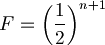# Coefficient of InbreedingThis article was checked by pedagogue, but more than year ago. Signature:  OSeda (talk)

 English: Coefficient of inbreedingCzech: koeficient inbreedinguCoefficient of inbreeding refers to the probability that an individual inherited both alleles of a particular locus / gene from a single ancestor. The coefficient is calculated as follows$F=\left(\frac{1}{2}\right)^{n+1}$

where n represents the number of steps in genealogy.